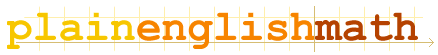You are here: MathematicsFundamentals → Relations

# Relations

The = sign called a relational operator, because it describes a relation between the two sides of the equation - in this case, the relation is equality.

But there are many other relational operators to choose from. Here are a few:

## Relational Operators

### ≡ Equivalence

Usage: LHS RHS
Using an equivalence sign instead of an equals sign in an equation, indicates that the expression on the left hand side (LHS) isn't just equal to the same number as the expression on the right hand side; rather, it's an alternative, equivalent way of writing the same thing.

The difference between "equal to" and "equivalent to" is sometimes very subtle. Generally you will be able to figure out when one is more appropriate than the other, but if in doubt, just use = instead.

### ≈ Approximation

Usage: LHSRHS
This means that the LHS and RHS are not strictly equal, but they are approximately equal, and the difference between them is too small to bother worrying about.

### ∝ Proportionality

Usage: LHS  RHS
The LHS is proportional to the RHS, meaning that the LHS is equal to the RHS multiplied by some constant - called a proportionality constant, which serves as a conversion factor between the LHS and RHS. For example, lengths in centimetres are proportional to their equivalent lengths in inches - you just have to multiply by 0.3937 to convert a quantity in centimetres to inches.

### ≠ Inequations

Usage: LHSRHS
This means that the LHS is not equal to the RHS. Inequations are the complete opposite of equations!

### ≤, ≥ Inequalities

 Usage: LHS ≤ RHS LHS ≥ RHS
≤ means "less than or equal to." The LHS is either equal to, or less than the RHS (i.e. left of the RHS on the number line).
Likewise, ≥ means "greater than or equal to." (i.e. LHS is either equal to, or to the right of RHS on the number line)

You can manipulate inequalities in the same way as equations - you can perform almost any operation on the inequality without breaking it, as long as you perform the same operation on both the LHS and RHS simultaneously. That is except for multiplication or division by a negative number! Think about it: multiplying any number by a negative scalar reverses the direction of that number. If it previously pointed in the positive direction, the multiplication will produce a negative number, and vice versa. Therefore, whenever you multiply or divide an inequality by a negative number, you must reverse the direction of the inequality symbol at the same time.

 For example: Take the following inequality: 5 ≥ -2 ← This is true Now multiplying both sides by -1 shouldn't change anything, right? -5 ≥ 2 ← Wrong!!! The inequality symbol now points the wrong way! This is better: -5 ≤ 2

### <, > Strict inequalities

Strict inequalities are pretty much identical to standard inequalities, except that this time, the LHS is strictly not equal to the RHS. < means "[strictly] less than," and > means "[strictly] greater than."